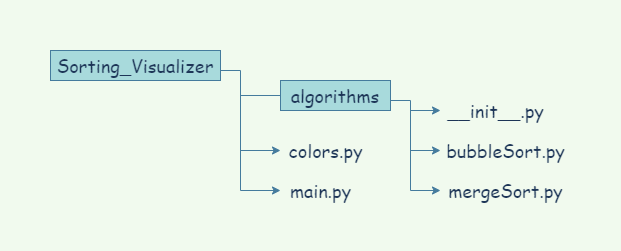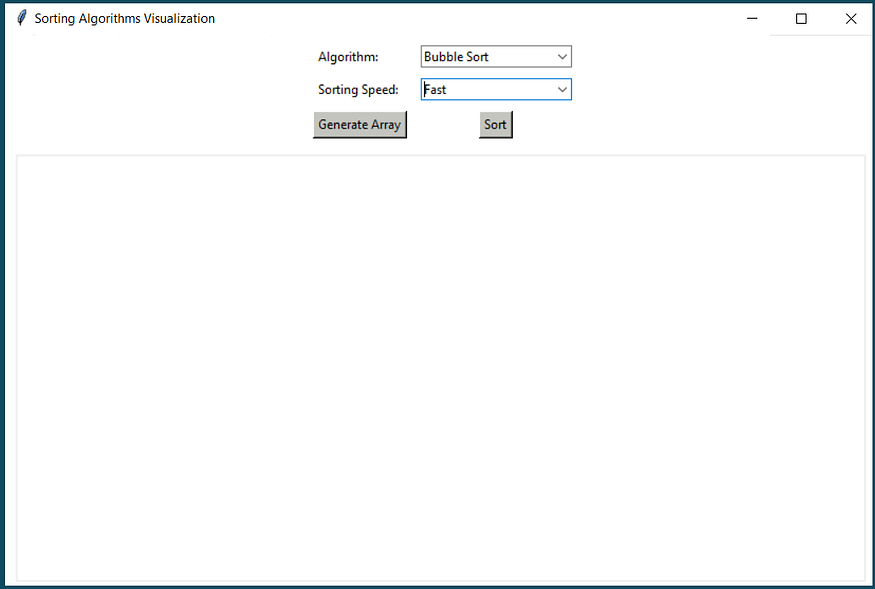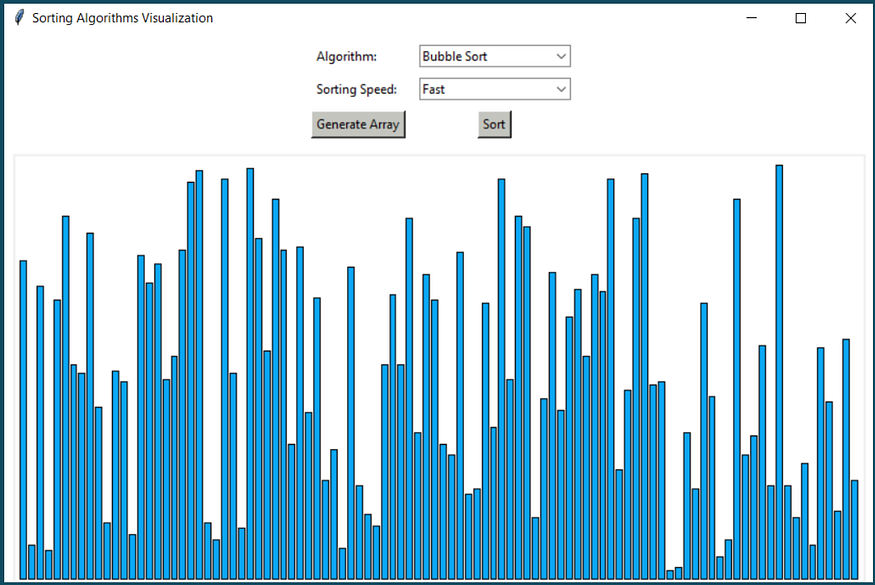# Build a Sorting Algorithm Visualizer in Python

## Create a simple GUI application using Python & Tkinter that visualizes various sorting algorithmsIn this article, we will build a Sorting Algorithm Visualizer using Python and Tkinter. Tkinter is a standard GUI library for Python. We will use it to create the user interface of our project. We will visualize Bubble Sort and Merge Sort. But we can implement all the comparison-based sorting algorithms in this project. Wikipedia page on comparison sort.

### File Structure of the Project

We will structure our project files at the very beginning. First, we will create a folder and name it `Sorting_Visualizer`. This will be our project directory. Inside this folder, we will create another folder named `algorithms`. Then we will create two python files `main.py` and `colors.py`. `main.py` will be our main Python file which we will execute. `colors.py` will contain some hexadecimal values of colors that we will need.

Inside the algorithm folder, we will create a file `__init__.py`. This will allow the `algorithms` folder to act as a Python package. See the documentation on packaging a project. We will create two more Python files inside the `algorithms` folder, `bubbleSort.py` and `mergeSort.py`. In these files, we will implement our sorting algorithms.

So our file structure will be like this…File structure for our project

### colors.py

In `colors.py` we will store some hexadecimal values of colors as variables. We will use these colors in our project.

``````DARK_GRAY = '#65696B'

LIGHT_GRAY = '#C4C5BF'

BLUE = '#0CA8F6'

DARK_BLUE = '#4204CC'

WHITE = '#FFFFFF'

BLACK = '#000000'

RED = '#F22810'

YELLOW = '#F7E806'

PINK = '#F50BED'

LIGHT_GREEN = '#05F50E'

PURPLE = '#BF01FB'
``````

### main.py

`main.py` will be our main executable file that will run our project. We will import all packages and modules in this file. First, we will import some stuff and set up a basic interface.

``````from tkinter import *

from tkinter import ttk

# We will need random to create array

import random

# Importing colors from colors.py that we made earlier

from colors import *

# Creating a basic window

window = Tk()

window.title("Sorting Algorithms Visualization")

window.maxsize(1000, 700)

window.config(bg = WHITE)

window.mainloop()
``````

Now we will add some variables and functions. We will edit the functions one by one. For now, we will just `pass`.

``````from tkinter import *

from tkinter import ttk

import random

from colors import *

# Main window

window = Tk()

window.title("Sorting Algorithms Visualization")

window.maxsize(1000, 700)

window.config(bg = WHITE)

algorithm_name = StringVar()

# algo_list is to select which alforithm we want to use to sort

algo_list = ['Bubble Sort', 'Merge Sort']

speed_name = StringVar()

# speed_list is for selecting sorting speed

speed_list = ['Fast', 'Medium', 'Slow']

# This empty list will be filled with random values every time we generate a new array

data = []

# This function will draw randomly generated list data[] on the canvas as vertical bars

def drawData(data, colorArray):

pass

# This function will generate array with random values every time we hit the generate button

def generate():

pass

# This function will set sorting speed

def set_speed():

pass

# This funciton will trigger a selected algorithm and start sorting

def sort():

pass

### User interface here ###

window.mainloop()
``````

Now we need a few things:

• Two dropdown menus, one to select the algorithm and one to select the sorting speed.
• Two buttons, one to generate the array and one to start sorting.
• A canvas to draw the array.

Let's add some code after the comment `### user interface here ###`.

``````UI_frame = Frame(window, width= 900, height=300, bg=WHITE)

# dropdown to select sorting algorithm

l1 = Label(UI_frame, text="Algorithm: ", bg=WHITE)

# dropdown to select sorting speed

l2 = Label(UI_frame, text="Sorting Speed: ", bg=WHITE)

# sort button

b1 = Button(UI_frame, text="Sort", command=sort, bg=LIGHT_GRAY)

# button for generating array

b3 = Button(UI_frame, text="Generate Array", command=generate, bg=LIGHT_GRAY)

# canvas to draw our array

canvas = Canvas(window, width=800, height=400, bg=WHITE)

``````

Now `main.py` will look like this…

``````from tkinter import *

from tkinter import ttk

import random

from colors import *

window = Tk()

window.title("Sorting Algorithms Visualization")

window.maxsize(1000, 700)

window.config(bg = WHITE)

algorithm_name = StringVar()

algo_list = ['Bubble Sort', 'Merge Sort']

speed_name = StringVar()

speed_list = ['Fast', 'Medium', 'Slow']

data = []

# This function will draw randomly generated list data[] on the canvas as vertical bars

def drawData(data, colorArray):

pass

# This function will generate array with random values every time we hit the generate button

def generate():

pass

# This function will set sorting speed

def set_speed():

pass

# This funciton will trigger a selected algorithm and start sorting

def sort():

pass

### User interface here ###

UI_frame = Frame(window, width= 900, height=300, bg=WHITE)

# dropdown to select sorting algorithm

l1 = Label(UI_frame, text="Algorithm: ", bg=WHITE)

# dropdown to select sorting speed

l2 = Label(UI_frame, text="Sorting Speed: ", bg=WHITE)

# sort button

b1 = Button(UI_frame, text="Sort", command=sort, bg=LIGHT_GRAY)

# button for generating array

b3 = Button(UI_frame, text="Generate Array", command=generate, bg=LIGHT_GRAY)

# canvas to draw our array

canvas = Canvas(window, width=800, height=400, bg=WHITE)

window.mainloop()
``````

When we run `main.py` we will get an output like the following:The basic UI

Our basic UI is done!

### Filling up The Functions

Now it's time to fill up the functions that we left empty before.

Let's start with `drawData()`. This function will convert the elements of `data[]` into vertical bars and draw them into the window.

``````def drawData(data, colorArray):

canvas.delete("all")

canvas_width = 800

canvas_height = 400

x_width = canvas_width / (len(data) + 1)

offset = 4

spacing = 2

normalizedData = [i / max(data) for i in data]

for i, height in enumerate(normalizedData):

x0 = i * x_width + offset + spacing

y0 = canvas_height - height * 390

x1 = (i + 1) * x_width + offset

y1 = canvas_height

canvas.create_rectangle(x0, y0, x1, y1, fill=colorArray[i])

``````

Next, we will write the `generate()` function which will create an array with random values with the help of `random` module. After creating the array we will store them in `data[]`. Then we will call the `drawData()` function from this function.

``````def generate():

global data

data = []

for i in range(0, 100):

random_value = random.randint(1, 150)

data.append(random_value)

drawData(data, [BLUE for x in range(len(data))])
``````

Now the program will create a random array on the window every time we hit the Generate Array button.Randomly generated array

Next, it's time to write the `set_speed()` function. This function will determine how fast or slow we want to see the sorting comparisons.

``````def set_speed():

return 0.3

return 0.1

else:

return 0.001
``````

Next, before we write the `sort()` function we have to write our sorting algorithms first. Then we will import the algorithms in `main.py`.

### Implementing Bubble Sort

Now we will go back to our `algorithms` folder. Open `bubbleSort.py` and write our bubble sort algorithm. We will create a function called `bubble_sort()`.

``````# We need the time module to create some time difference between each comparison

import time

# Importing colors from colors.py

from colors import *

def bubble_sort(data, drawData, timeTick):

size = len(data)

for i in range(size-1):

for j in range(size-i-1):

if data[j] > data[j+1]:

data[j], data[j+1] = data[j+1], data[j]

drawData(data, [YELLOW if x == j or x == j+1 else BLUE for x in range(len(data))] )

time.sleep(timeTick)

drawData(data, [BLUE for x in range(len(data))])
``````

### Implementing Merge Sort

We will implement merge sort similarly we implemented bubble sort. From `algorithms` folder we will open `mergeSort.py` and write our `merge_sort()` function.

``````import time

from colors import *

def merge(data, start, mid, end, drawData, timeTick):

p = start

q = mid + 1

tempArray = []

for i in range(start, end+1):

if p > mid:

tempArray.append(data[q])

q+=1

elif q > end:

tempArray.append(data[p])

p+=1

elif data[p] < data[q]:

tempArray.append(data[p])

p+=1

else:

tempArray.append(data[q])

q+=1

for p in range(len(tempArray)):

data[start] = tempArray[p]

start += 1

def merge_sort(data, start, end, drawData, timeTick):

if start < end:

mid = int((start + end) / 2)

merge_sort(data, start, mid, drawData, timeTick)

merge_sort(data, mid+1, end, drawData, timeTick)

merge(data, start, mid, end, drawData, timeTick)

drawData(data, [PURPLE if x >= start and x < mid else YELLOW if x == mid

else DARK_BLUE if x > mid and x <=end else BLUE for x in range(len(data))])

time.sleep(timeTick)

drawData(data, [BLUE for x in range(len(data))])
``````

### Completing what we left behind

Now we will go back to `main.py`. We will import the algorithms. Then we will complete the `sort()` function.

To import the algorithms we have to put the following two lines on top of `main.py`.

``````from algorithms.bubbleSort import bubble_sort

from algorithms.mergeSort import merge_sort
``````

Next, it's time to complete the `sort()` function.

``````def sort():

global data

timeTick = set_speed()

bubble_sort(data, drawData, timeTick)

merge_sort(data, 0, len(data)-1, drawData, timeTick)
``````

Finally `main.py` is completed! Here is the finalized `main.py`.

``````from tkinter import *

from tkinter import ttk

import random

from colors import *

# Importing algorithms

from algorithms.bubbleSort import bubble_sort

from algorithms.mergeSort import merge_sort

# Main window

window = Tk()

window.title("Sorting Algorithms Visualization")

window.maxsize(1000, 700)

window.config(bg = WHITE)

algorithm_name = StringVar()

algo_list = ['Bubble Sort', 'Merge Sort']

speed_name = StringVar()

speed_list = ['Fast', 'Medium', 'Slow']

data = []

# This function will draw randomly generated list data[] on the canvas as vertical bars

def drawData(data, colorArray):

canvas.delete("all")

canvas_width = 800

canvas_height = 400

x_width = canvas_width / (len(data) + 1)

offset = 4

spacing = 2

normalizedData = [i / max(data) for i in data]

for i, height in enumerate(normalizedData):

x0 = i * x_width + offset + spacing

y0 = canvas_height - height * 390

x1 = (i + 1) * x_width + offset

y1 = canvas_height

canvas.create_rectangle(x0, y0, x1, y1, fill=colorArray[i])

# This function will generate array with random values every time we hit the generate button

def generate():

global data

data = []

for i in range(0, 100):

random_value = random.randint(1, 150)

data.append(random_value)

drawData(data, [BLUE for x in range(len(data))])

# This function will set sorting speed

def set_speed():

return 0.3

return 0.1

else:

return 0.001

# This funciton will trigger a selected algorithm and start sorting

def sort():

global data

timeTick = set_speed()

bubble_sort(data, drawData, timeTick)

merge_sort(data, 0, len(data)-1, drawData, timeTick)

### User interface here ###

UI_frame = Frame(window, width= 900, height=300, bg=WHITE)

# dropdown to select sorting algorithm

l1 = Label(UI_frame, text="Algorithm: ", bg=WHITE)

# dropdown to select sorting speed

l2 = Label(UI_frame, text="Sorting Speed: ", bg=WHITE)

# sort button

b1 = Button(UI_frame, text="Sort", command=sort, bg=LIGHT_GRAY)

# button for generating array

b3 = Button(UI_frame, text="Generate Array", command=generate, bg=LIGHT_GRAY)

# canvas to draw our array

canvas = Canvas(window, width=800, height=400, bg=WHITE)

window.mainloop()
``````

Now when we run `main.py` the sorting will work and we will be able to visualize the algorithms.

Here is a short demo of the project.

Project demo

### Find the Project on GitHub

I implemented seven different sorting algorithms in this project. Here is the GitHub repository of the project.

And there you have it! A fun and cool project to sharpen your Python and algorithms skills. I hope you find the article helpful. Thanks for reading.